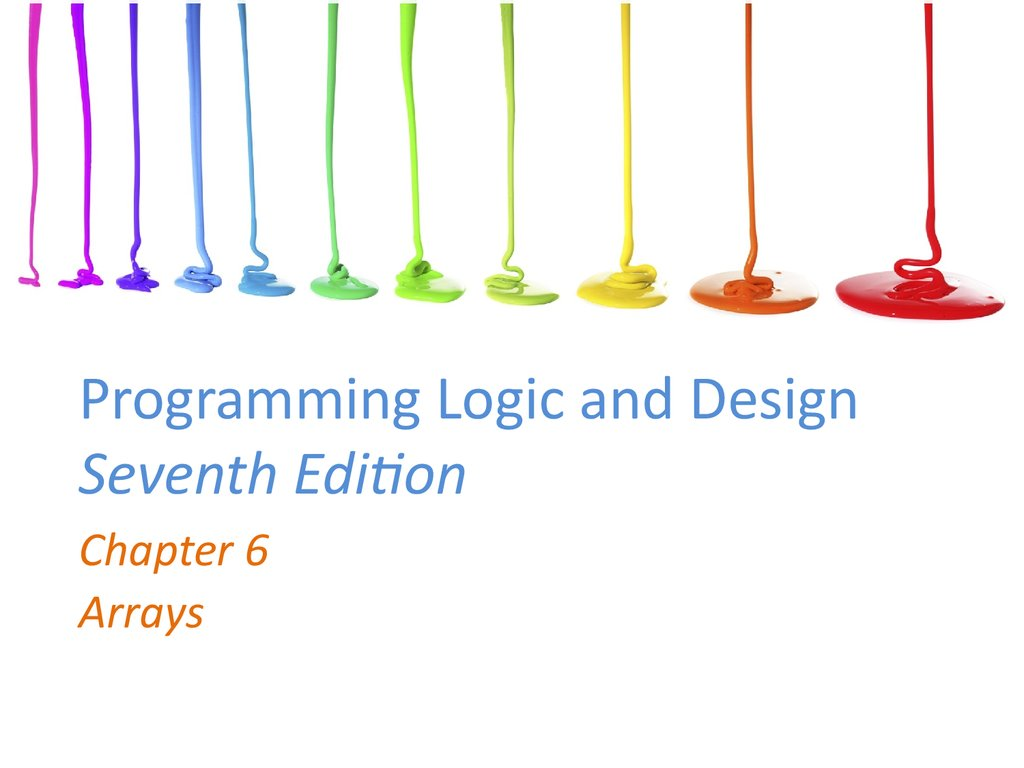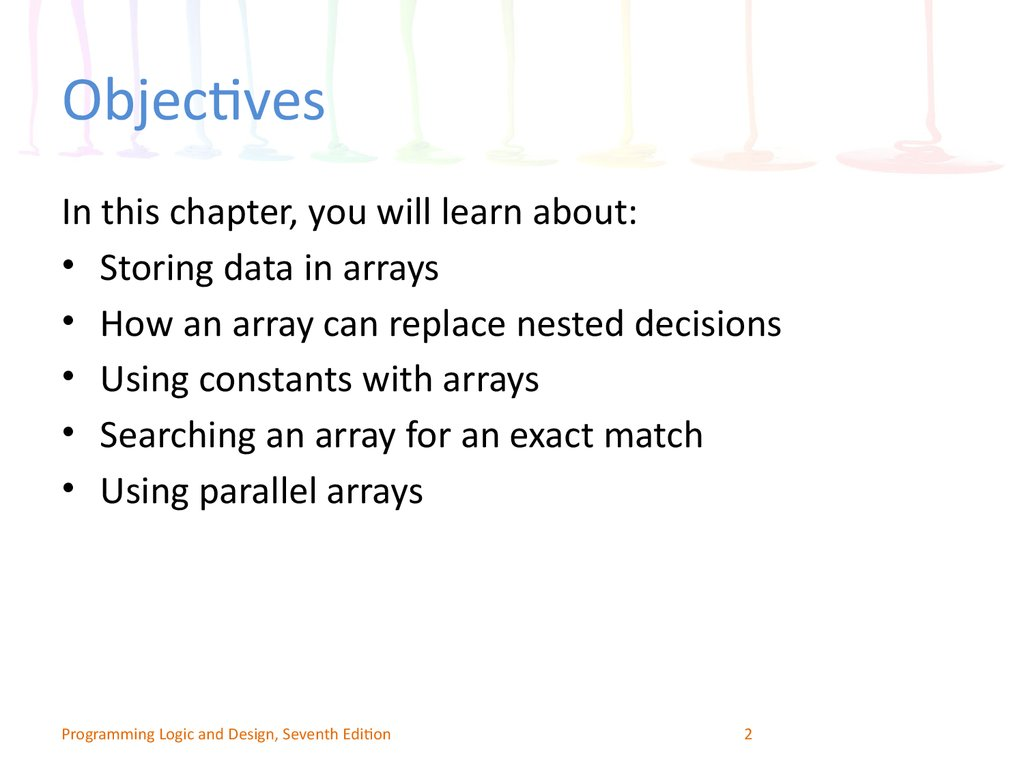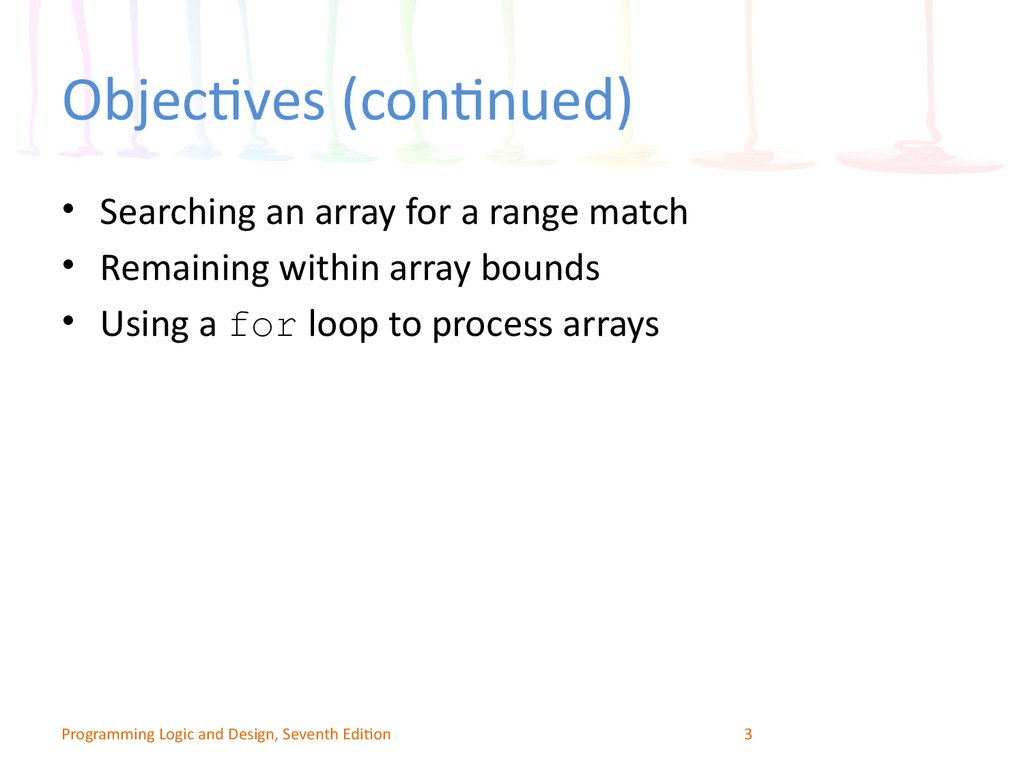# Programming Logic and Design Seventh Edition

Chapter 6
Arrays

## 2. Objectives

In this chapter, you will learn about:
• Storing data in arrays
• How an array can replace nested decisions
• Using constants with arrays
• Searching an array for an exact match
• Using parallel arrays
Programming Logic and Design, Seventh Edition
2

## 3. Objectives (continued)

• Searching an array for a range match
• Remaining within array bounds
• Using a for loop to process arrays
Programming Logic and Design, Seventh Edition
3

## 4. How Arrays Occupy Computer Memory

• Array
– A series or list of variables in computer memory
– All variables share the same name
– Each variable has a different subscript
• Subscript (or index)
– Position number of an item in an array
– Subscripts are always a sequence of integers
Programming Logic and Design, Seventh Edition
4

## 5.

How Arrays Occupy Computer
Memory (continued)
• Each item has the same name and the same data type
• Element: an item in the array
– Array elements are contiguous in memory
• Size of the array: the number of elements it will hold
• Subscripts or indexes: indicate the position of a
particular element in the array
• Adding data values is called populating the array
Programming Logic and Design, Seventh Edition
5

## 6. How Arrays Occupy Computer Memory (continued)

Figure 6-1 Appearance of a three-element array in computer memory
Programming Logic and Design, Seventh Edition
6

## 7. How an Array Can Replace Nested Decisions

• Example: Human Resources Department Dependents
report
– List employees who have claimed zero through five
dependents
• Assume no employee has more than five dependents
• Application produces counts for dependent
categories
– Uses a series of decisions
• Application does not scale up to more dependents
Programming Logic and Design, Seventh Edition
7

## 8.

Figure 6-3 Flowchart and pseudocode of decision-making process using a series
of decisions—the hard way
Programming Logic and Design, Seventh Edition
8

## 9. How an Array Can Replace Nested Decisions (continued)

• The array reduces the number of statements needed
• Six dependent count accumulators are redefined as a
single array
• Variable as a subscript to the array
• Array subscript variable must be:
– Numeric with no decimal places
– Initialized to 0
– Incremented by 1 each time the logic passes through the
loop
Programming Logic and Design, Seventh Edition
9

## 10.

Figure 6-4 Flowchart and pseudocode of decision-making process—but still the hard way
Programming Logic and Design, Seventh Edition
10

## 11.

Figure 6-5 Flowchart and pseudocode of decision-making process
using an array—but still a hard way
Programming Logic and Design, Seventh Edition
11

## 12. How an Array Can Replace Nested Decisions (continued)

Figure 6-6 Flowchart and pseudocode of efficient decision-making
process using an array
Programming Logic and Design, Seventh Edition
12

## 13.

Figure 6-7 Flowchart and pseudocode for Dependents report program
Programming Logic and Design, Seventh Edition
13

## 14. How an Array Can Replace Nested Decisions (continued)

Figure 6-7 Flowchart and pseudocode for Dependents report program (continued)
Programming Logic and Design, Seventh Edition
14

## 15. Using Constants with Arrays

• Use constants in several ways
– To hold the size of an array
– As the array element values
– As an array subscript
Programming Logic and Design, Seventh Edition
15

## 16. Using a Constant as the Size of an Array

• Avoid “magic numbers” (unnamed constants)
• Declare a named numeric constant to be used every
time the array is accessed
• Make sure any subscript remains less than the
constant value
• Constants are created automatically in many
languages
Programming Logic and Design, Seventh Edition
16

## 17. Using Constants as Array Element Values

• Sometimes the values stored in arrays should be
constants
• Example
string MONTH = "January",
"February", "March", "April", "May",
"June", "July", "August", "September",
"October", "November", "December"
Programming Logic and Design, Seventh Edition
17

## 18. Using a Constant as an Array Subscript

• Use a numeric constant as a subscript to an array
• Example
– Declare a named constant as: num INDIANA = 5
– Display value with:
output salesArray[INDIANA]
Programming Logic and Design, Seventh Edition
18

## 19. Searching an Array for an Exact Match

• Sometimes you must search through an entire array to
find a value

Item numbers are three-digit, non-consecutive numbers
Customer orders an item; check if item number is valid
Create an array that holds valid item numbers
Search the array for an exact match
Programming Logic and Design, Seventh Edition
19

## 20.

Figure 6-8 Flowchart and pseudocode for a program that verifies item availability
Programming Logic and Design, Seventh Edition
20

## 21.

Figure 6-8 Flowchart and pseudocode for a program that verifies item availability (continued)
Programming Logic and Design, Seventh Edition
21

## 22.

Figure 6-8 Flowchart and pseudocode for a program that verifies item availability (continued)
Programming Logic and Design, Seventh Edition
22

## 23. Searching an Array for an Exact Match (continued)

• Flag: a variable that indicates whether an event
occurred
• Technique for searching an array
– Set a subscript variable to 0 to start at the first element
– Initialize a flag variable to false to indicate the desired value
has not been found
– Examine each element in the array
– If the value matches, set the flag to True
– If the value does not match, increment the subscript and
examine the next array element
Programming Logic and Design, Seventh Edition
23

## 24. Using Parallel Arrays

– Two arrays, each with six elements
• Valid item numbers
• Valid item prices
– Each price in the valid item price array is in the same position as
the corresponding item in the valid item number array
• Parallel arrays
– Each element in one array is associated with an element in the
same relative position in the other array
• Look through the valid item array for the customer’s item
– When a match is found, get the price from the item price array
Programming Logic and Design, Seventh Edition
24

## 25. Using Parallel Arrays (continued)

Figure 6-9 Parallel arrays in memory
Programming Logic and Design, Seventh Edition
25

## 26. Using Parallel Arrays (continued)

• Use parallel arrays
– Two or more arrays contain related data
– A subscript relates the arrays
• Elements at the same position in each array are logically related
• Indirect relationship
– Relationship between an item’s number and its price
– Parallel arrays are very useful
Programming Logic and Design, Seventh Edition
26

## 27.

Figure 6-10 Flowchart and pseudocode of a program that finds an item price using
parallel arrays
Programming Logic and Design, Seventh Edition
27

## 28.

Figure 6-10 Flowchart and pseudocode of a program that finds an item price using
parallel arrays (continued)
Programming Logic and Design, Seventh Edition
28

## 29.

Figure 6-10 Flowchart and pseudocode of a program that finds an item price using
parallel arrays (continued)
Programming Logic and Design, Seventh Edition
29

## 30. Improving Search Efficiency

• The program should stop searching the array when
a match is found
• Set a variable to a specific value instead of letting
normal processing set it
• Improves efficiency
• The larger the array, the better the improvement by
doing an early exit
Programming Logic and Design, Seventh Edition
30

## 31.

Figure 6-11 Flowchart and pseudocode of the module that finds an item price and
exits the loop as soon as it is found
Programming Logic and Design, Seventh Edition
31

## 32. Improving Search Efficiency (continued)

Figure 6-11 Flowchart and pseudocode of the module that finds an item price and
exits the loop as soon as it is found (continued)
Programming Logic and Design, Seventh Edition
32

## 33. Searching an Array for a Range Match

• Programmers may want to work with ranges of
values in arrays, 1 through 5 or 20 through 30
– Read the customer order data; determine the discount
based on the quantity ordered
• First approach
– An array with as many elements as each possible order
quantity
– Store the appropriate discount for each possible order
quantity
Programming Logic and Design, Seventh Edition
33

## 34. Searching an Array for a Range Match (continued)

Figure 6-13 Usable—but inefficient—discount array
Programming Logic and Design, Seventh Edition
34

## 35. Searching an Array for a Range Match (continued)

• Drawbacks of first approach
– Requires a very large array; uses a lot of memory
– Stores the same value repeatedly
– How do you know when you have enough elements?
• Customer can always order more
• Better approach
– Create four discount array elements for each discount
rate
– A parallel array with a discount range
• Use a loop to make comparisons
Programming Logic and Design, Seventh Edition
35

## 36. Searching an Array for a Range Match (continued)

Figure 6-14 Parallel arrays to use for determining discount
Programming Logic and Design, Seventh Edition
36

## 37.

Figure 6-15 Program that determines discount rate
Programming Logic and Design, Seventh Edition
37

## 38. Remaining within Array Bounds

• Every array has a finite size
– Number of elements in the array
– Number of bytes in the array
• Arrays are composed of elements of the same data type
• Elements of the same data type occupy the same
number of bytes in memory
• The number of bytes in an array is always a multiple of
the number of array elements
• Access data using a subscript containing a value that
accesses memory occupied by the array
Programming Logic and Design, Seventh Edition
38

## 39. Remaining within Array Bounds (continued)

Figure 6-16 Determining the month string from user’s numeric entry
Programming Logic and Design, Seventh Edition
39

## 40. Remaining within Array Bounds (continued)

• Program logic assumes every number entered by
the user is valid
• When an invalid subscript is used:
– Some languages stop execution and issue an error
– Other languages access a memory location outside of the
array
• An invalid array subscript is a logical error
• Out of bounds: using a subscript that is not within
the acceptable range for the array
• The program should prevent bounds errors
Programming Logic and Design, Seventh Edition
40

## 41. Using a for Loop to Process Arrays

• for loop: a single statement
– Initializes the loop control variable
– Compares it to a limit
– Alters it
• The for loop is especially convenient when
working with arrays
– To process every element
• Must stay within array bounds
• Highest usable subscript is one less than the array
size
Programming Logic and Design, Seventh Edition
41

## 42. Using a for Loop to Process Arrays (continued)

Figure 6-17 Pseudocode that uses a for loop to display an array of department names
Programming Logic and Design, Seventh Edition
42

## 43. Using a for Loop to Process Arrays (continued)

Figure 6-18 Pseudocode that uses a more efficient for loop to output department names
Programming Logic and Design, Seventh Edition
43

## 44. Summary

• Array: a named series or list of values in memory
– Same data type
– Different subscript
• Use a variable as a subscript to the array to replace
multiple nested decisions
• Constants can be used to hold an array’s size
• Searching through an array requires
– Initializing a subscript
– Using a loop to test each element
– Setting a flag when a match is found
Programming Logic and Design, Seventh Edition
44

## 45. Summary (continued)

• Parallel arrays: each element in one array is
associated with the element in a second array
– Elements have the same relative position
• For range comparisons, store either the low- or
high-end value of each range
Programming Logic and Design, Seventh Edition
45

## 46. Summary (continued)

• Access data in an array
– Use a subscript containing a value that accesses memory
occupied by the array
• A subscript is out of bounds if it is not within the
defined range of acceptable subscripts
• The for loop is a convenient tool for working with
arrays
– Process each element of an array from beginning to end
Programming Logic and Design, Seventh Edition
46